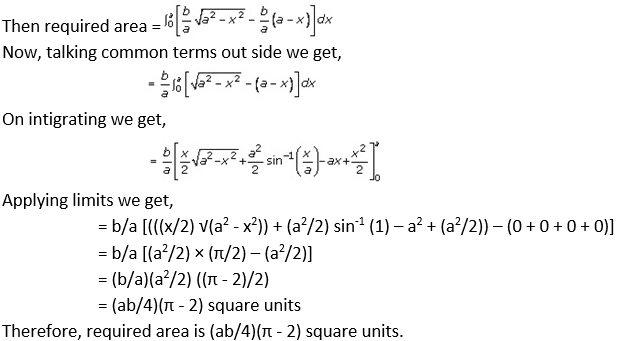# RD Sharma Solutions Class 12 Area Bounded Regions Exercise 21.3

RD Sharma Solutions for Class 12 Maths Exercise 21.3 Chapter 21 Areas of Bounded Regions are available here. We, at BYJU’S, ensure that you get the best RD Sharma Solutions for Class 12 Maths Chapter 21 study material that can help you excel in your studies, with a well-organized way of learning. Your difficulties are addressed rightly, empowering you to achieve excellent marks in your class and exams.

## Download PDF of RD Sharma Solutions for Class 12 Maths Chapter 21 Exercise 3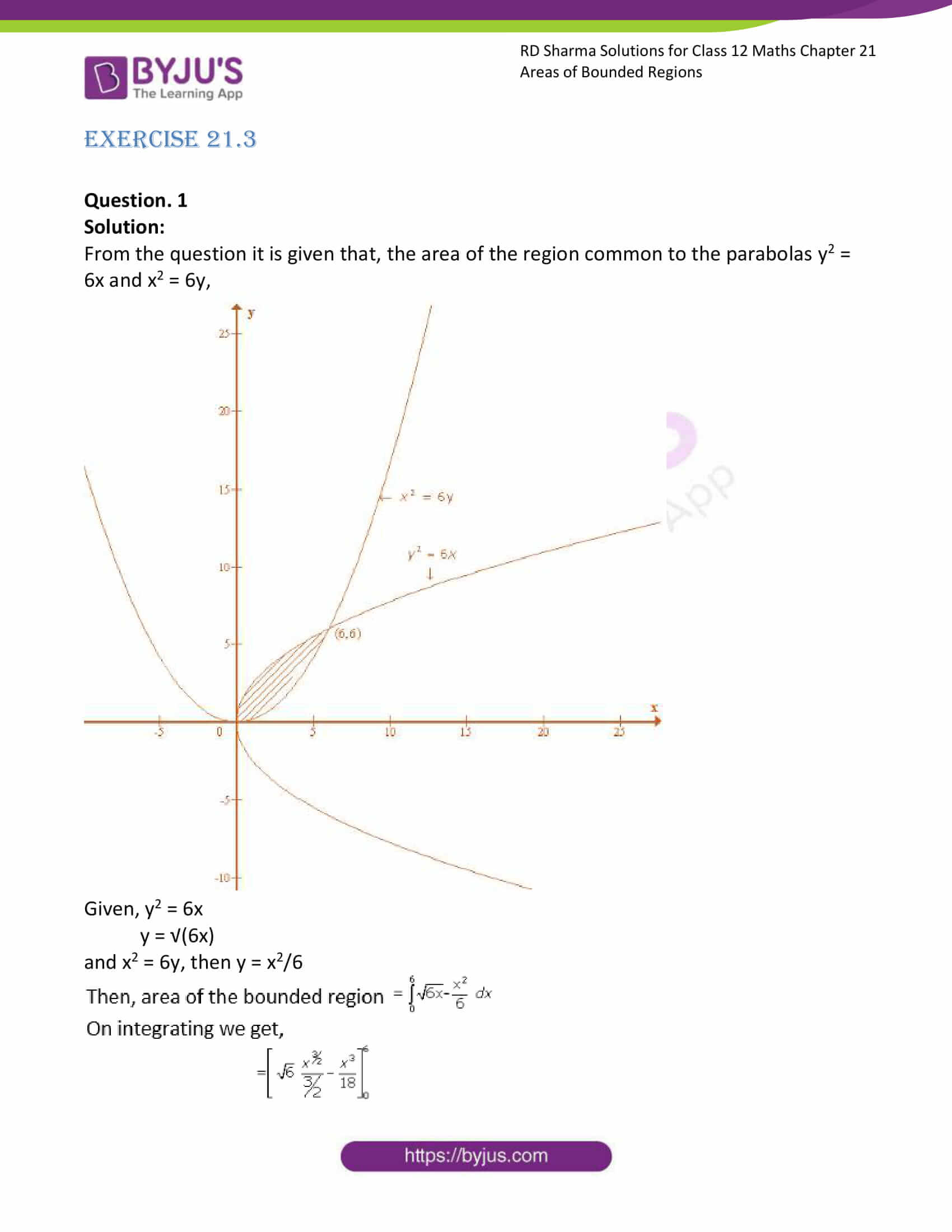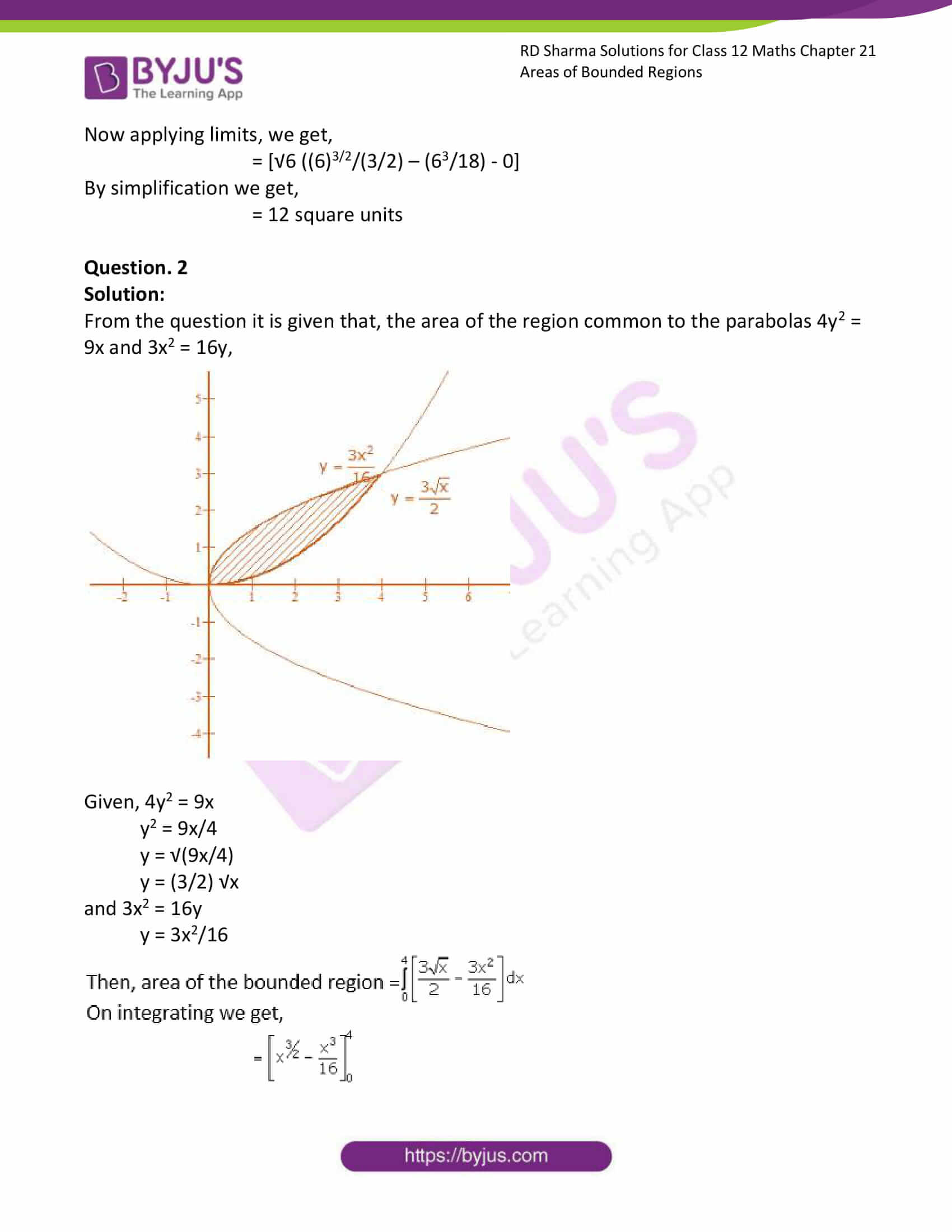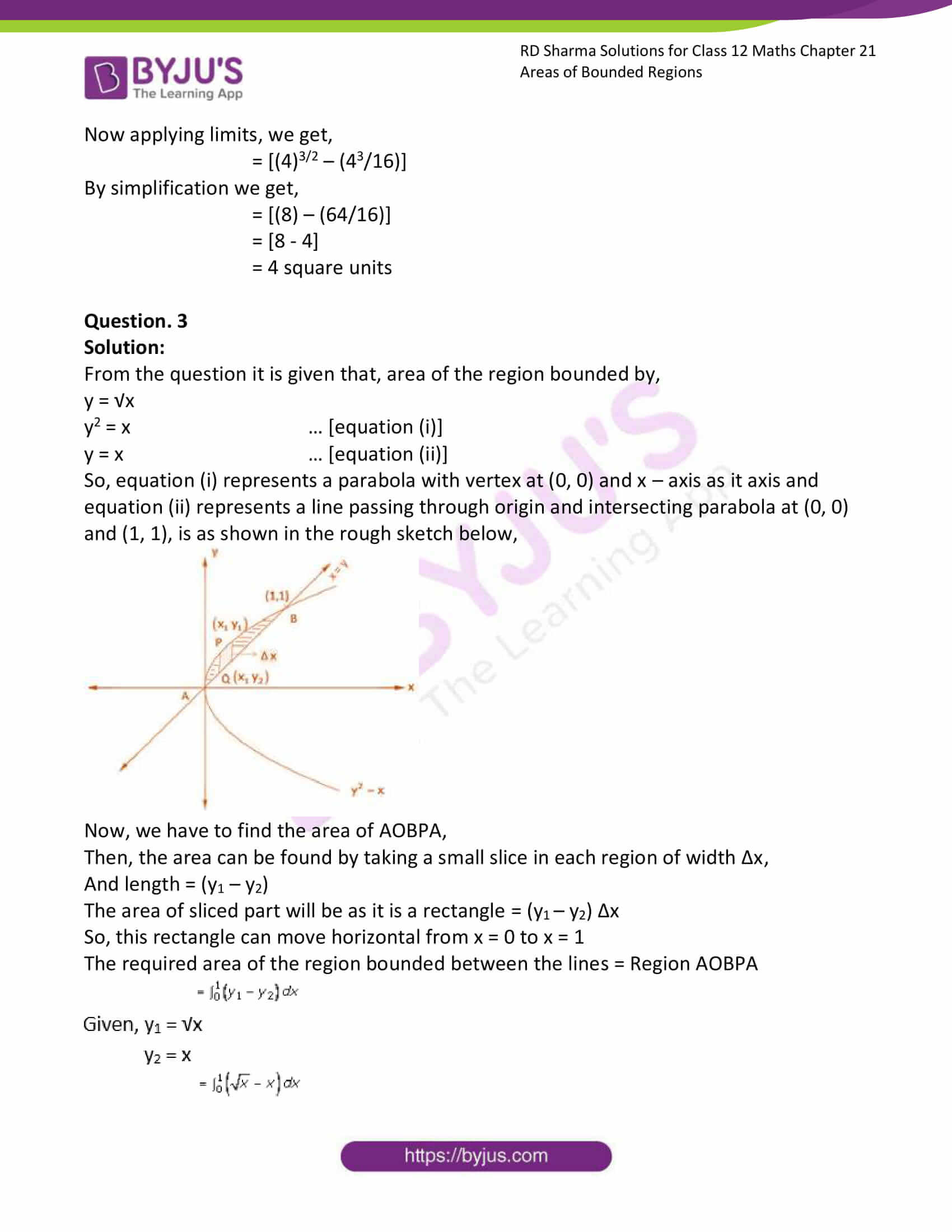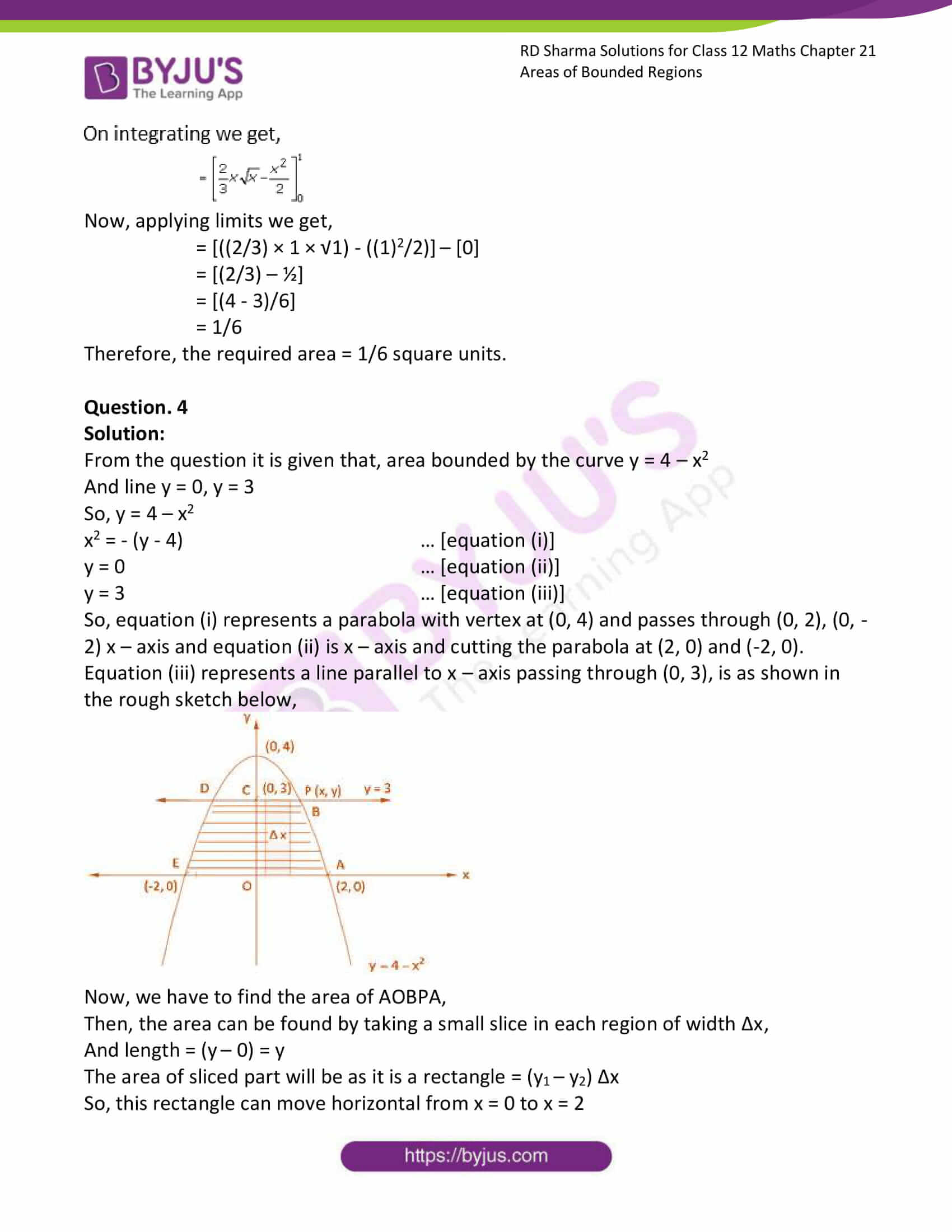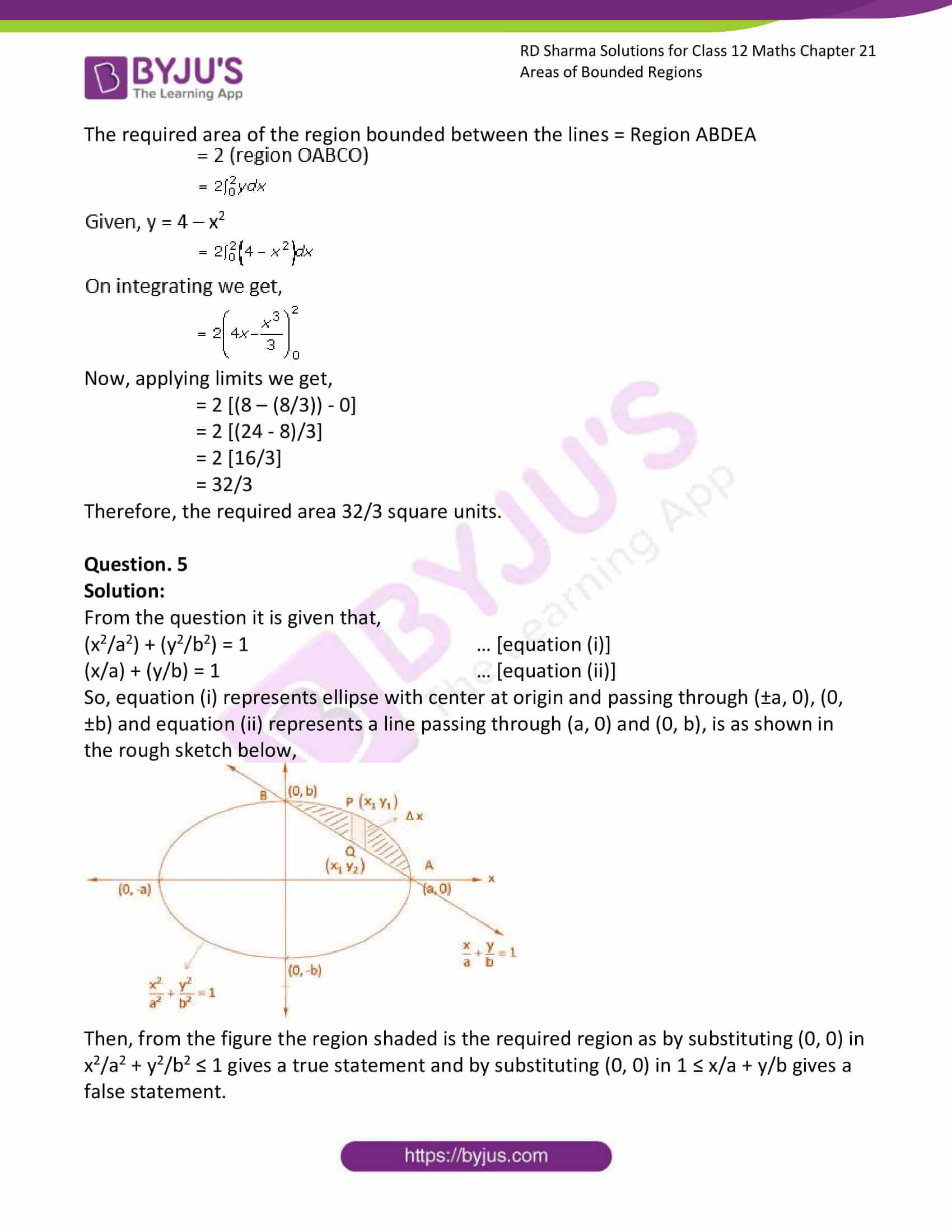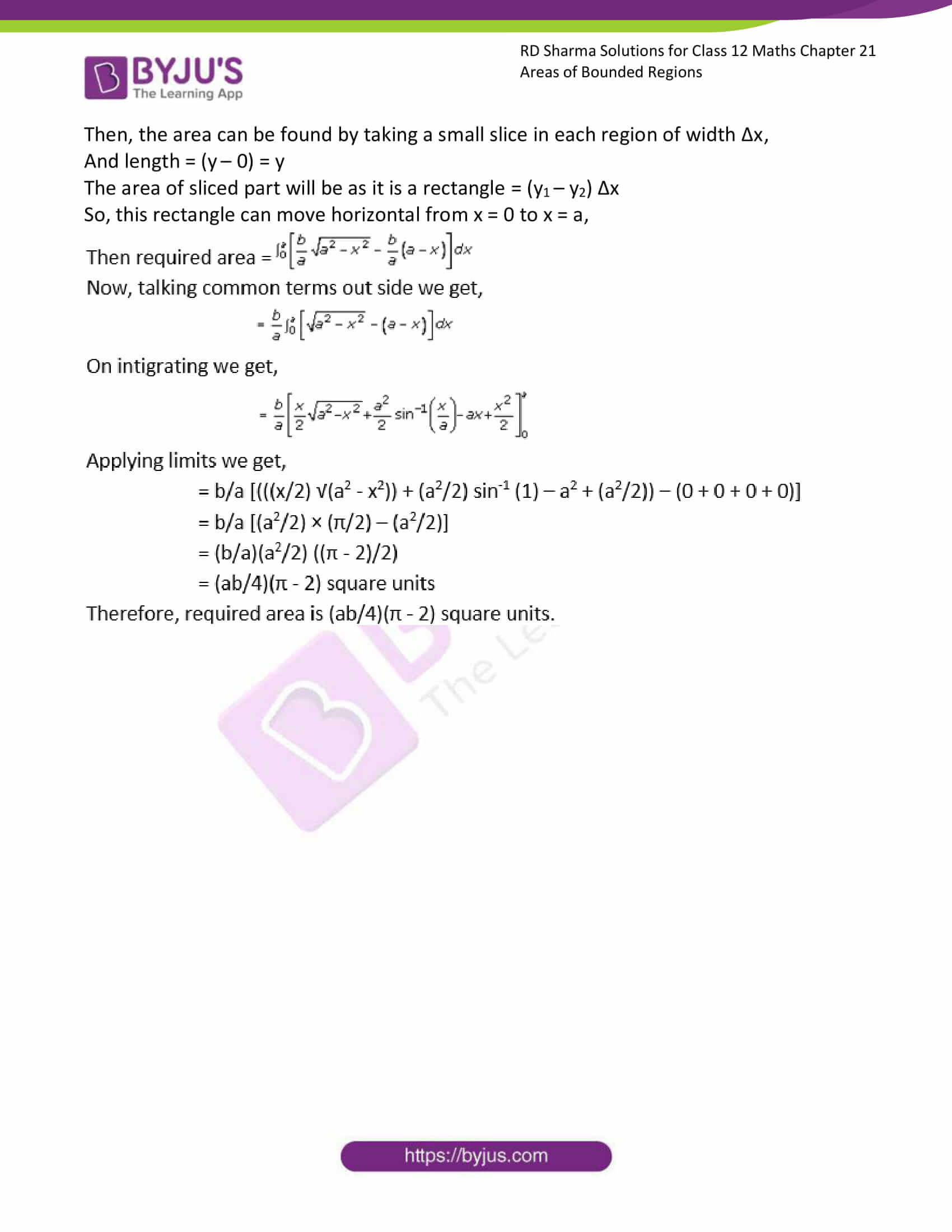### Access RD Sharma Solutions for Class 12 Maths Chapter 21 Exercise 3

EXERCISE 21.3

Question. 1

Solution:

From the question it is given that, the area of the region common to the parabolas y2 = 6x and x2 = 6y,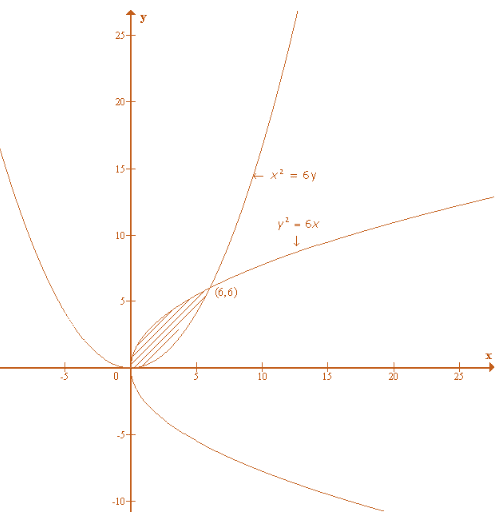Given, y2 = 6x

y = √(6x)

and x2 = 6y, then y = x2/6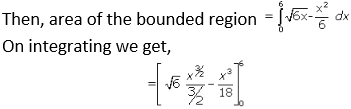Now applying limits, we get,

= [√6 ((6)3/2/(3/2) – (63/18) – 0]

By simplification we get,

= 12 square units

Question. 2

Solution:

From the question it is given that, the area of the region common to the parabolas 4y2 = 9x and 3x2 = 16y,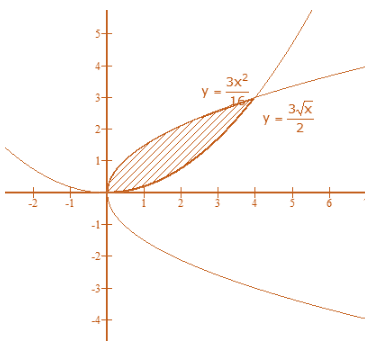Given, 4y2 = 9x

y2 = 9x/4

y = √(9x/4)

y = (3/2) √x

and 3x2 = 16y

y = 3x2/16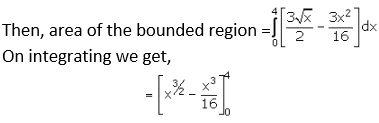Now applying limits, we get,

= [(4)3/2 – (43/16)]

By simplification we get,

= [(8) – (64/16)]

= [8 – 4]

= 4 square units

Question. 3

Solution:

From the question it is given that, area of the region bounded by,

y = √x

y2 = x … [equation (i)]

y = x … [equation (ii)]

So, equation (i) represents a parabola with vertex at (0, 0) and x – axis as it axis and equation (ii) represents a line passing through origin and intersecting parabola at (0, 0) and (1, 1), is as shown in the rough sketch below,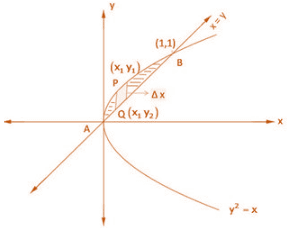Now, we have to find the area of AOBPA,

Then, the area can be found by taking a small slice in each region of width Δx,

And length = (y1 – y2)

The area of sliced part will be as it is a rectangle = (y1 – y2) Δx

So, this rectangle can move horizontal from x = 0 to x = 1

The required area of the region bounded between the lines = Region AOBPA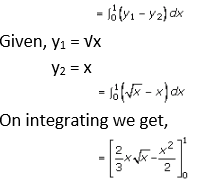Now, applying limits we get,

= [((2/3) × 1 × √1) – ((1)2/2)] – 

= [(2/3) – ½]

= [(4 – 3)/6]

= 1/6

Therefore, the required area = 1/6 square units.

Question. 4

Solution:

From the question it is given that, area bounded by the curve y = 4 – x2

And line y = 0, y = 3

So, y = 4 – x2

x2 = – (y – 4) … [equation (i)]

y = 0 … [equation (ii)]

y = 3 … [equation (iii)]

So, equation (i) represents a parabola with vertex at (0, 4) and passes through (0, 2), (0, -2) x – axis and equation (ii) is x – axis and cutting the parabola at (2, 0) and (-2, 0). Equation (iii) represents a line parallel to x – axis passing through (0, 3), is as shown in the rough sketch below,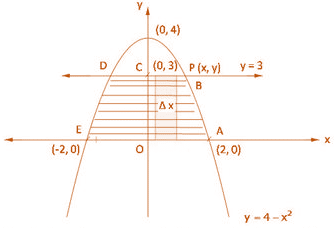Now, we have to find the area of AOBPA,

Then, the area can be found by taking a small slice in each region of width Δx,

And length = (y – 0) = y

The area of sliced part will be as it is a rectangle = (y1 – y2) Δx

So, this rectangle can move horizontal from x = 0 to x = 2

The required area of the region bounded between the lines = Region ABDEA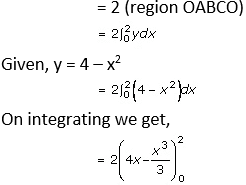Now, applying limits we get,

= 2 [(8 – (8/3)) – 0]

= 2 [(24 – 8)/3]

= 2 [16/3]

= 32/3

Therefore, the required area 32/3 square units.

Question. 5

Solution:

From the question it is given that,

(x2/a2) + (y2/b2) = 1 … [equation (i)]

(x/a) + (y/b) = 1 … [equation (ii)]

So, equation (i) represents ellipse with center at origin and passing through (±a, 0), (0, ±b) and equation (ii) represents a line passing through (a, 0) and (0, b), is as shown in the rough sketch below,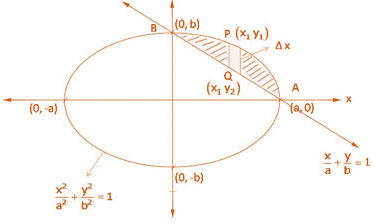Then, from the figure the region shaded is the required region as by substituting (0, 0) in x2/a2 + y2/b2 ≤ 1 gives a true statement and by substituting (0, 0) in 1 ≤ x/a + y/b gives a false statement.

Then, the area can be found by taking a small slice in each region of width Δx,

And length = (y – 0) = y

The area of sliced part will be as it is a rectangle = (y1 – y2) Δx

So, this rectangle can move horizontal from x = 0 to x = a,